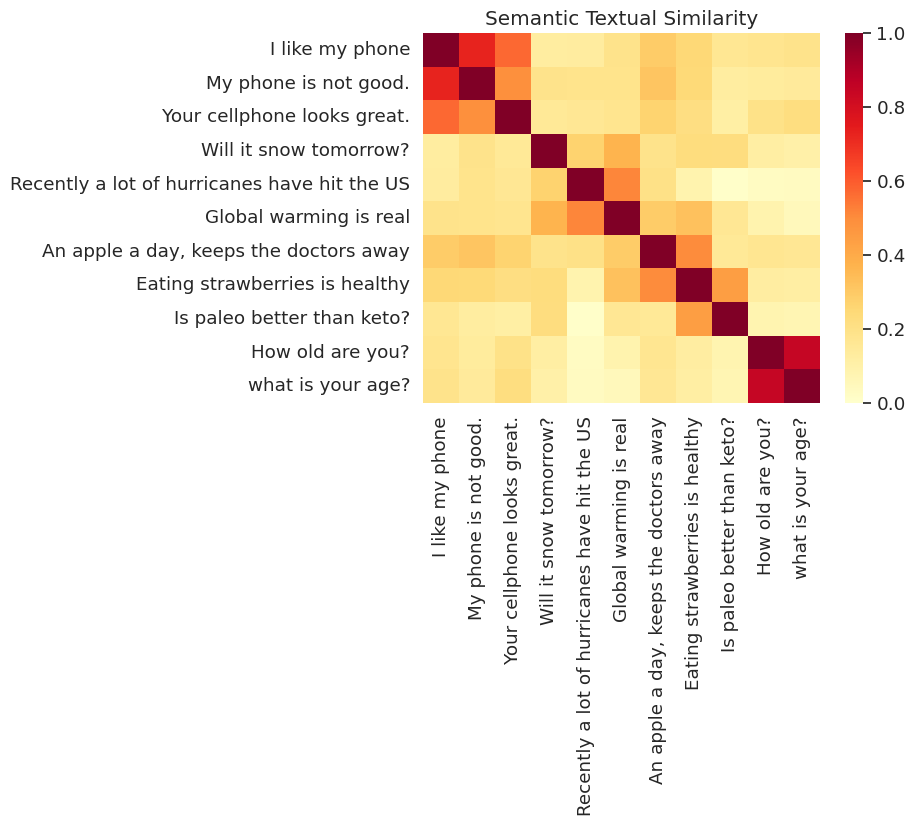# Universal Sentence Encoder-Lite 演示

Universal Sentence Encoder 使获取句子级别的嵌入向量变得与以往查找单个单词的嵌入向量一样容易。之后，您可以轻松地使用句子嵌入向量计算句子级别的语义相似度，以及使用较少监督的训练数据在下游分类任务中实现更好的性能。

# 开始

## 设置

````# Install seaborn for pretty visualizations`
`pip3 install --quiet seaborn`
`# Install SentencePiece package`
`# SentencePiece package is needed for Universal Sentence Encoder Lite. We'll`
`# use it for all the text processing and sentence feature ID lookup.`
`pip3 install --quiet sentencepiece`
```
``````from absl import logging

import tensorflow.compat.v1 as tf
tf.disable_v2_behavior()

import tensorflow_hub as hub
import sentencepiece as spm
import matplotlib.pyplot as plt
import numpy as np
import os
import pandas as pd
import re
import seaborn as sns
``````
```WARNING:tensorflow:From /tmpfs/src/tf_docs_env/lib/python3.7/site-packages/tensorflow/python/compat/v2_compat.py:101: disable_resource_variables (from tensorflow.python.ops.variable_scope) is deprecated and will be removed in a future version.
Instructions for updating:
non-resource variables are not supported in the long term
```

## 从 TF-Hub 加载模块

``````module = hub.Module("https://hub.tensorflow.google.cn/google/universal-sentence-encoder-lite/2")
``````
``````input_placeholder = tf.sparse_placeholder(tf.int64, shape=[None, None])
encodings = module(
inputs=dict(
values=input_placeholder.values,
indices=input_placeholder.indices,
dense_shape=input_placeholder.dense_shape))
``````
```INFO:tensorflow:Saver not created because there are no variables in the graph to restore
INFO:tensorflow:Saver not created because there are no variables in the graph to restore
```

## 从 TF-Hub 模块加载 SentencePiece 模型

SentencePiece 模型方便地存储在模块的资源中。您必须加载它才能初始化处理器。

``````with tf.Session() as sess:
spm_path = sess.run(module(signature="spm_path"))

sp = spm.SentencePieceProcessor()
with tf.io.gfile.GFile(spm_path, mode="rb") as f:
``````
```INFO:tensorflow:Saver not created because there are no variables in the graph to restore
INFO:tensorflow:Saver not created because there are no variables in the graph to restore
```
``````def process_to_IDs_in_sparse_format(sp, sentences):
# An utility method that processes sentences with the sentence piece processor
# 'sp' and returns the results in tf.SparseTensor-similar format:
# (values, indices, dense_shape)
ids = [sp.EncodeAsIds(x) for x in sentences]
max_len = max(len(x) for x in ids)
dense_shape=(len(ids), max_len)
values=[item for sublist in ids for item in sublist]
indices=[[row,col] for row in range(len(ids)) for col in range(len(ids[row]))]
return (values, indices, dense_shape)
``````

### 使用一些样本测试模块

``````# Compute a representation for each message, showing various lengths supported.
word = "Elephant"
sentence = "I am a sentence for which I would like to get its embedding."
paragraph = (
"Universal Sentence Encoder embeddings also support short paragraphs. "
"There is no hard limit on how long the paragraph is. Roughly, the longer "
"the more 'diluted' the embedding will be.")
messages = [word, sentence, paragraph]

values, indices, dense_shape = process_to_IDs_in_sparse_format(sp, messages)

# Reduce logging output.
logging.set_verbosity(logging.ERROR)

with tf.Session() as session:
session.run([tf.global_variables_initializer(), tf.tables_initializer()])
message_embeddings = session.run(
encodings,
feed_dict={input_placeholder.values: values,
input_placeholder.indices: indices,
input_placeholder.dense_shape: dense_shape})

for i, message_embedding in enumerate(np.array(message_embeddings).tolist()):
print("Message: {}".format(messages[i]))
print("Embedding size: {}".format(len(message_embedding)))
message_embedding_snippet = ", ".join(
(str(x) for x in message_embedding[:3]))
print("Embedding: [{}, ...]\n".format(message_embedding_snippet))
``````
```Message: Elephant
Embedding size: 512
Embedding: [0.053387489169836044, 0.053194381296634674, -0.052356015890836716, ...]

Message: I am a sentence for which I would like to get its embedding.
Embedding size: 512
Embedding: [0.03533298149704933, -0.04714975506067276, 0.012305550277233124, ...]

Message: Universal Sentence Encoder embeddings also support short paragraphs. There is no hard limit on how long the paragraph is. Roughly, the longer the more 'diluted' the embedding will be.
Embedding size: 512
Embedding: [-0.004081667400896549, -0.08954868465662003, 0.03737196698784828, ...]
```

# 语义文本相似度 (STS) 任务示例

Universal Sentence Encoder 生成的嵌入向量会被近似归一化。两个句子的语义相似度可以作为编码的内积轻松进行计算。

``````def plot_similarity(labels, features, rotation):
corr = np.inner(features, features)
sns.set(font_scale=1.2)
g = sns.heatmap(
corr,
xticklabels=labels,
yticklabels=labels,
vmin=0,
vmax=1,
cmap="YlOrRd")
g.set_xticklabels(labels, rotation=rotation)
g.set_title("Semantic Textual Similarity")

def run_and_plot(session, input_placeholder, messages):
values, indices, dense_shape = process_to_IDs_in_sparse_format(sp,messages)

message_embeddings = session.run(
encodings,
feed_dict={input_placeholder.values: values,
input_placeholder.indices: indices,
input_placeholder.dense_shape: dense_shape})

plot_similarity(messages, message_embeddings, 90)
``````

## 可视化相似度

``````messages = [
# Smartphones
"I like my phone",
"My phone is not good.",

# Weather
"Will it snow tomorrow?",
"Recently a lot of hurricanes have hit the US",
"Global warming is real",

# Food and health
"An apple a day, keeps the doctors away",
"Eating strawberries is healthy",
"Is paleo better than keto?",

"How old are you?",
]

with tf.Session() as session:
session.run(tf.global_variables_initializer())
session.run(tf.tables_initializer())
run_and_plot(session, input_placeholder, messages)
``````## 评估：STS（语义文本相似度）基准

STS 基准会根据从句子嵌入向量计算得出的相似度得分与人为判断的一致程度，提供内部评估。该基准要求系统为不同的句子对选择返回相似度得分。然后使用皮尔逊相关来评估机器相似度得分相对于人为判断的质量。

### 下载数据

``````import pandas
import scipy
import math

# Loads a subset of the STS dataset into a DataFrame. In particular both
# sentences and their human rated similarity score.
sent_pairs = []
with tf.gfile.GFile(filename, "r") as f:
for line in f:
ts = line.strip().split("\t")
# (sent_1, sent_2, similarity_score)
sent_pairs.append((ts, ts, float(ts)))
return pandas.DataFrame(sent_pairs, columns=["sent_1", "sent_2", "sim"])

sts_dataset = tf.keras.utils.get_file(
fname="Stsbenchmark.tar.gz",
origin="http://ixa2.si.ehu.es/stswiki/images/4/48/Stsbenchmark.tar.gz",
extract=True)

os.path.join(os.path.dirname(sts_dataset), "stsbenchmark", "sts-dev.csv"))
os.path.join(
os.path.dirname(sts_dataset), "stsbenchmark", "sts-test.csv"))

return sts_dev, sts_test

``````

### 构建评估计算图

``````sts_input1 = tf.sparse_placeholder(tf.int64, shape=(None, None))
sts_input2 = tf.sparse_placeholder(tf.int64, shape=(None, None))

# For evaluation we use exactly normalized rather than
# approximately normalized.
sts_encode1 = tf.nn.l2_normalize(
module(
inputs=dict(values=sts_input1.values,
indices=sts_input1.indices,
dense_shape=sts_input1.dense_shape)),
axis=1)
sts_encode2 = tf.nn.l2_normalize(
module(
inputs=dict(values=sts_input2.values,
indices=sts_input2.indices,
dense_shape=sts_input2.dense_shape)),
axis=1)

sim_scores = -tf.acos(tf.reduce_sum(tf.multiply(sts_encode1, sts_encode2), axis=1))
``````
```INFO:tensorflow:Saver not created because there are no variables in the graph to restore
INFO:tensorflow:Saver not created because there are no variables in the graph to restore
INFO:tensorflow:Saver not created because there are no variables in the graph to restore
INFO:tensorflow:Saver not created because there are no variables in the graph to restore
```

### Choose dataset for benchmark

``````def run_sts_benchmark(session):
"""Returns the similarity scores"""
scores = session.run(
sim_scores,
feed_dict={
sts_input1.values: values1,
sts_input1.indices:  indices1,
sts_input1.dense_shape:  dense_shape1,
sts_input2.values:  values2,
sts_input2.indices:  indices2,
sts_input2.dense_shape:  dense_shape2,
})
return scores

with tf.Session() as session:
session.run(tf.global_variables_initializer())
session.run(tf.tables_initializer())
scores = run_sts_benchmark(session)

pearson_correlation = scipy.stats.pearsonr(scores, similarity_scores)
print('Pearson correlation coefficient = {0}\np-value = {1}'.format(
pearson_correlation, pearson_correlation))
``````
```Pearson correlation coefficient = 0.7856484874001958
p-value = 1.065794746e-314
```
[]
[]